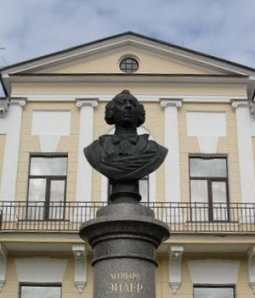#Euler Institute Program Cohomology in Mathematics and Physics

The program is to be held at the Euler Institute from September 2 until October 18, 2013

## General description

Though the notion of cohomology is fundamental to modern mathematics, its importance was not seen for some 40 years after the development of homology. The idea of cohomology can be traced back to the fundamental work of H. Poincare on dual cell structure in 1895. In 1935 A. Kolmogorov and J. Aleksander introduced the cohomology; the algebraic notion of a cocycle, introduced by D. K. Faddeev, S. Eilenberg and S. Mac Lane in 1943, became the foundation for homological algebra. In quantum physics, cohomology appeared at the end of 1960's in one-loop computations for gauge theory. Nowadays cohomology theories play a fundamental role both in mathematics, from algebraic topology to number theory, and in physics, from condensed matter theory to quantum gauge theories and string theory.

The goal of present program is to expose Russian and foreign mathematicians and theoretical physicists to the frontier of research in above topics. In order to achieve this it is proposed to have introductory lectures, more advanced mini-courses as well as two workshops and concluding conference. Main emphasis will be the introduction of Russian participants, mostly graduate students and young researchers, into this fundamental branch of mathematics and theoretical physics.